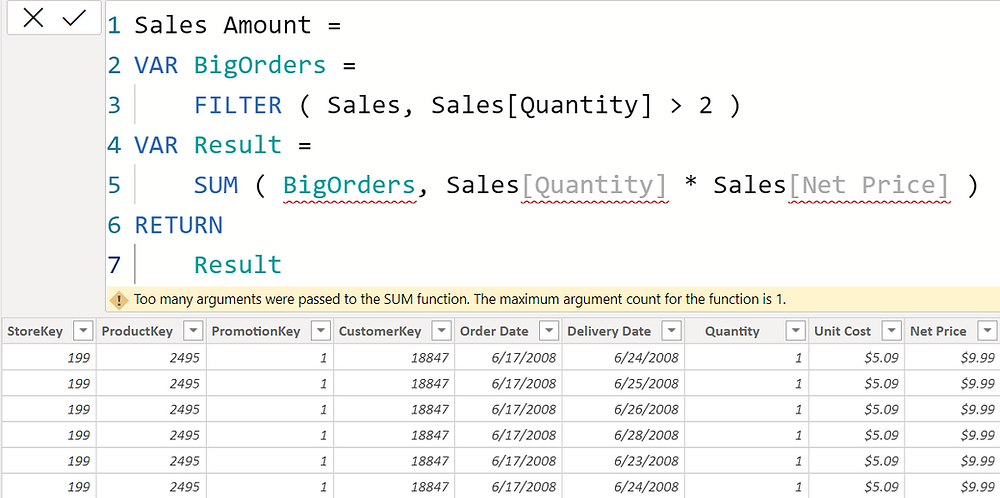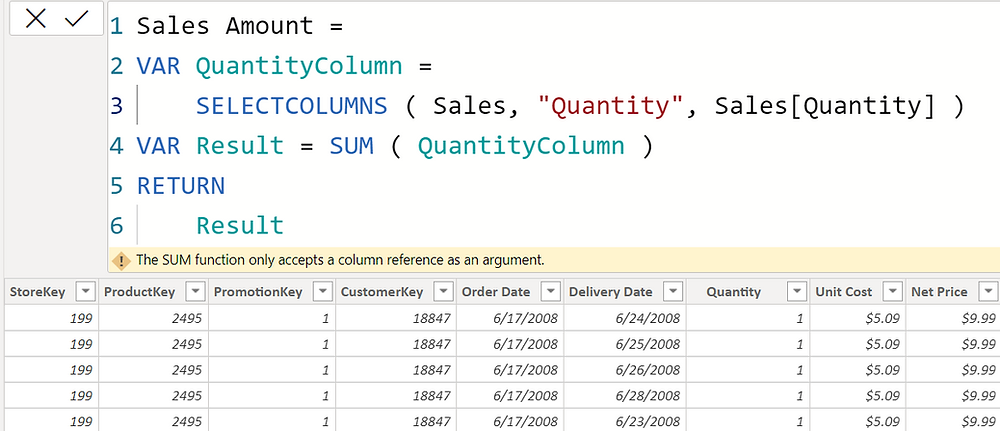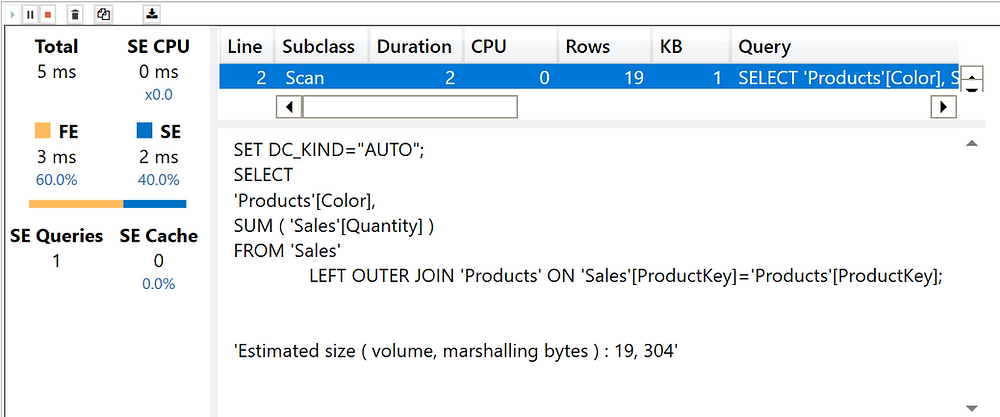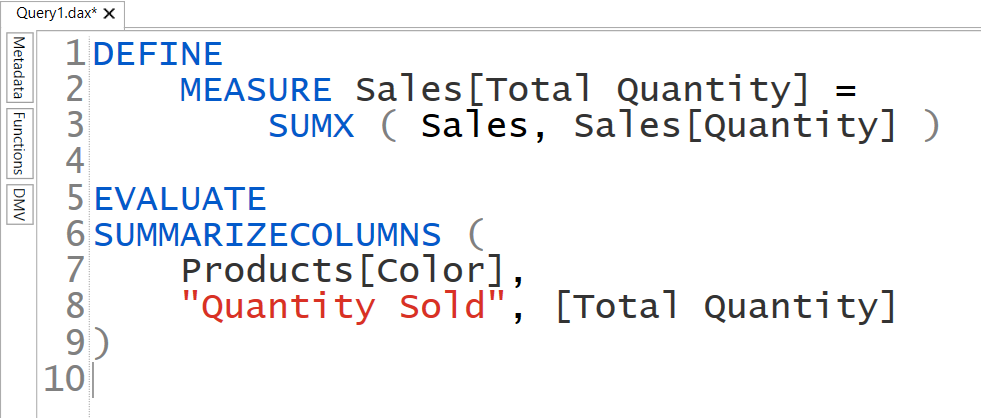Search
•Antriksh Sharma

# SUM vs SUMX in DAX

SUM and SUMX are both aggregation functions in DAX. While SUM allows you to aggregate only one Physical column of a table, SUMX is more flexible and allows Tables, Physical or Virtual Columns, and Variable references as well as complex expressions in row context.

Some(read sum, lol!) examples of SUM and SUMX before we get to the gist of the blog:

1. Summing a single column:

If you want to SUM a single column then you can choose between either SUM or SUMX.

Let's say I want to aggregate the quantity column in my Sales table.I can use SUM ( Sales[Quantity] ) or SUMX ( Sales, Sales[Quantity] )

2. Summing multiple columns:

Let's say I want to calculate the Revenue/Sales Amount by multiplying Quantity and Net price, now this is where things get tricky, since SUM can only except one argument, I can only use SUMX here.

Using SUM throws an error:SUMX works fine:3. Using variables

Let's say I want to find out the sales where the Quantity sold is greater than 2.

SUM will not work because it can only take a column reference and we can't supply a variable reference to it:With SUMX I can distribute the code into variables and then later use a variable in the first argument of SUMXWith SUM if I try to use a variable of single column, it still won't work:SUM doesn't Iterates:

Now that the difference between SUM and SUMX is established, let's take a look at a common statement that I have heard from a lot of people, which is that SUM is an aggregator but SUMX is an iterator.

To be honest the above claim is true but only for explaining the difference and teaching someone DAX. But behind the scenes everything in DAX is an iterator and I am not saying this because I feel it, you can actually verify this yourself by opening DAX Studio and turning of the Query Plan option.

I have opened DAX Studio and turned on both Query Plan and Server Timings option.Now let's create a simple query that will SUM Quantity by the Product[Color].

I have created a Total Quantity measure in DAX Studio that is going to exist only for the duration of the DAX Studio's session. And I am slicing the sum of Quantity by Product Color.Now if I execute this code by pressing F5, I get this result:So far everything is good, let's take a loot at the Server Timings tab to understand what DAX engine internally had to do to give us back this result:The above image shows us that the Storage Engine executed 1 query which fetched Total Quantity grouped by Products[Color] a SQL equivalent would be

SELECT

Color,

SUM(Quantity) AS [Quantity Sold]

FROM

Sales AS S

LEFT JOIN Products AS P

ON P.ProductKey = S.ProductKey

By looking at Storage Engine queries you can always find out how a code is internally executed by the DAX engines.

Now let's examine the Storage Engine query, when I change SUM to SUMX.

Replaced the code of SUM with SUMX equivalent.This time the Storage Engine query is still the same:So, one thing is clear from Storage Engine query that internally both SUM and SUMX will execute the same query when used on a single column.

Now let's move to the Query Plan tab to examine Logical and Physical Query Plan of SUM.

In DAX first a Logical Query plan is built by the Formula Engine, which defines a structure in which certain steps are to be performed by the Storage Engine and then a Physical Query Plan is fired when it is time to return the result

With the help of Logical Query Plan you can understand the flow of the code:In the above image you can see that:

1. One Line 2 we have Scan_Vertipaq operator which Scan Vertipaq (Storage Engine) for extracting the Products[Color] column.

2. On Line 3 we have a sibling operation which is supposed to do a SUM inside the Storage Engine, and for performing this operation we have 2 child operations, first the engine does a Scan_Vertipaq operation to get Products[Color] and Sales[Quantity] and then it is further used for processing and summing once this is done, the Sum_Vertipaq part is complete.

3. After all of these operations are complete, on Line 1 we see GroupSemiJoin, which is like INNER JOIN is SQL, this operation simply joins the data cache containing Product[Color] and [Quantity Sold]

In Physical Query Plan we get to see more detailed information such as:

1. Cache, which is extracted using a Storage Engine query that we saw earlier.

2. Then we have a ProjectionSpool, which simply hold the data cache in a temporarily in memory,

3. and before that we see Spool_Iterator which basically iterates the Data Cache and returns the result.If I execute the SUMX version, then also the query plan is same as can be seen from the image below:So it doesn't matter if you use SUM or SUMX, at the end of the day the following happens.

Formula Engine receives the DAX code, it convert it into set of instructions, which are then passed to Storage Engine which returns result to Formula Engine in form of uncompressed Data Caches, Formula Engine then iterates those Data Caches, in complex code, Formula Engine has to perform several operations between 2 or more data caches. Once Formula engine is done with its part, the result is return to the user in form of a measure or a table.

So next time you hear someone say that SUM is Faster than SUMX or SUM is an aggregator and SUMX is an iterator just know that behind the scenes both are the same. And the same is applicable to AVERAGE vs AVERAGEX, MIN vs MINX, MAX vs MAX and other pairs or any other DAX function.

## Recent Posts

See All

#### Tabular Editor 3 Course

TE3 is the ultimate development tool for creating Tabular Model and recently I got an opportunity to make a full fledged course on it for Enterprise DNA. Here is a post about that and how you can secu